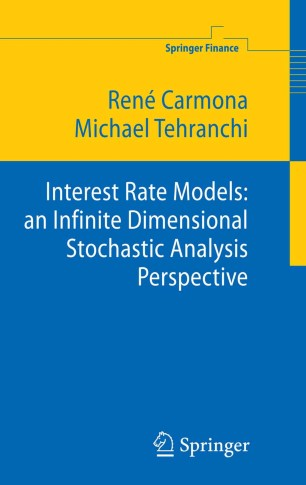# Interest Rate Models: an Infinite Dimensional Stochastic Analysis Perspective

• René A. Carmona
• Michael R. TehranchiBook

Part of the Springer Finance book series (FINANCE)

1. Front Matter
Pages I-XIV
2. ### The Term Structure of Interest Rates

1. Front Matter
Pages 1-1
2. Pages 43-72
3. ### Infinite Dimensional Stochastic Analysis

1. Front Matter
Pages 73-73
2. Pages 75-100
3. Pages 101-133
4. Pages 135-159
4. ### Generalized Models for the Term Structure of Interest Rates

1. Front Matter
Pages 161-161
2. Pages 163-194
3. Pages 195-215
5. Back Matter
Pages 217-235

### Introduction

Interest Rate Models: an Infinite Dimensional Stochastic Analysis Perspective studies the mathematical issues that arise in modeling the interest rate term structure. These issues are approached by casting the interest rate models as stochastic evolution equations in infinite dimensions. The book is comprised of three parts. Part I is a crash course on interest rates, including a statistical analysis of the data and an introduction to some popular interest rate models. Part II is a self-contained introduction to infinite dimensional stochastic analysis, including SDE in Hilbert spaces and Malliavin calculus. Part III presents some recent results in interest rate theory, including finite dimensional realizations of HJM models, generalized bond portfolios, and the ergodicity of HJM models.

### Keywords

Infinite-Dimensional Stochastic Analysis Interest-Rate Models Malliavin Calculus calculus ergodicity modeling statistical analysis

#### Authors and affiliations

• René A. Carmona
• 1
• Michael R. Tehranchi
• 2
1. 1.Bendheim Center for Finance Department of Operations Research and Financial EngineeringPrinceton UniversityPrincetonUSA
2. 2.Statistical Laboratory Centre for Mathematical SciencesUniversity of CambridgeCambridgeUK